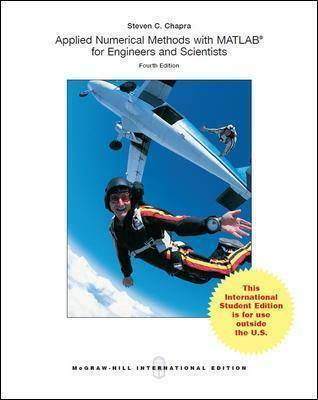# APPLIED NUMERICAL METHOD WTIH MATLAB FOR ENGINEERS AND SCIENTISTS 4E - STEVEN CHAPRA 9781260083255

RM 120.00

TITLE :APPLIED NUMERICAL METHOD WTIH MATLAB FOR ENGINEERS AND SCIENTISTS - STEVEN CHAPRA

ISBN13 : 9781260083255

PUBLISHER : MCGRAWHILL (2018)

EDITION : 4E ISE PAPERBACK

PAGES :  736 PAGES

Applied Numerical Methods with MATLAB is written for students who want to learn and apply numerical methods in order to solve problems in engineering and science. As such, the methods are motivated by problems rather than by mathematics. That said, sufficient theory is provided so that students come away with insight into the techniques and their shortcomings.

Part One - Modeling, Computers, and Error Analysis

1) Mathematical Modeling, Numerical Methods, and Problem Solving

2) MATLAB Fundamentals

3) Programming with MATLAB

4) Roundoff and Truncation Errors

Part Two - Roots and Optimization

5) Roots: Bracketing Methods

6) Roots: Open Methods

7) Optimization

Part Three - Linear Systems

8) Linear Algebraic Equations and Matrices

9) Gauss Elimination

10) LU Factorization

11) Matrix Inverse and Condition

12) Iterative Methods

13) Eigenvalues

Part Four - Curve Fitting

14) Linear Regression

15) General Linear Least-Squares and Nonlinear Regression

16) Fourier Analysis

17) Polynomial Interpolation

18) Splines and Piecewise Interpolation

Part Five - Integration and Differentiation

19) Numerical Integration Formulas

20) Numerical Integration of Functions

21) Numerical Differentiation

Part Six - Ordinary Differential Equations

22) Initial-Value Problems

23) Adaptive Methods and Stiff Systems

24) Boundary-Value Problems

Appendix A - MATLAB Built-In Functions

Appendix B - MATLAB M-File Functions

Index